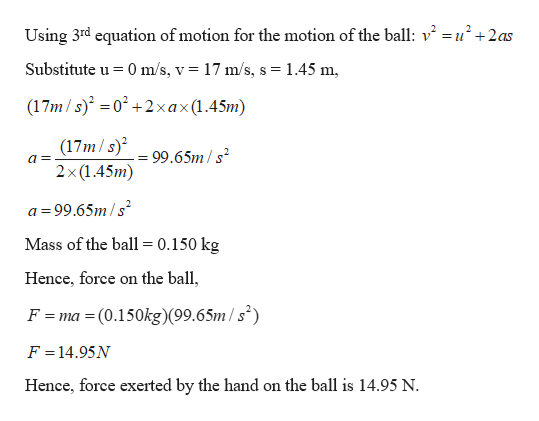# As a protest against the umpire's calls, a baseball pitcher throws a ball straight up into the air at a speed of 17.0 m/s. In the process, he moves his hand through a distance of 1.45 m. If the ball has a mass of 0.150 kg, find the force he exerts on the ball to give it this upward speed.N

Question

As a protest against the umpire's calls, a baseball pitcher throws a ball straight up into the air at a speed of 17.0 m/s. In the process, he moves his hand through a distance of 1.45 m. If the ball has a mass of 0.150 kg, find the force he exerts on the ball to give it this upward speed.
N

check_circle

Step 1

Given:

Mass of the ball = 0.150 kg

Distance moved by the ball before leaving from the hand = 1.45 m

Intial velocity of the ball = 0 m/s

Final velocity of the ball = 17 m/s

Step 2

Calculating the force exerted b...help_outlineImage TranscriptioncloseUsing 3rd equation of motion for the motion of the ball: v2 =u2+2as Substitute u 0 m/s, v 17 m/s, s 1.45 m (17m/s) 02+2xax(1.45m) (17m/s) 2x(1.45m) 99.65m/s a= = a 99.65m/s Mass of the ball = 0.150 kg Hence, force on the ball, F = ma = (0.150kg)(99.6 5m / s°) F = 14.95N Hence, force exerted by the hand on the ball is 14.95 N fullscreen

### Want to see the full answer?

See Solution

#### Want to see this answer and more?

Solutions are written by subject experts who are available 24/7. Questions are typically answered within 1 hour.*

See Solution
*Response times may vary by subject and question.
Tagged in

### Newtons Laws of Motion